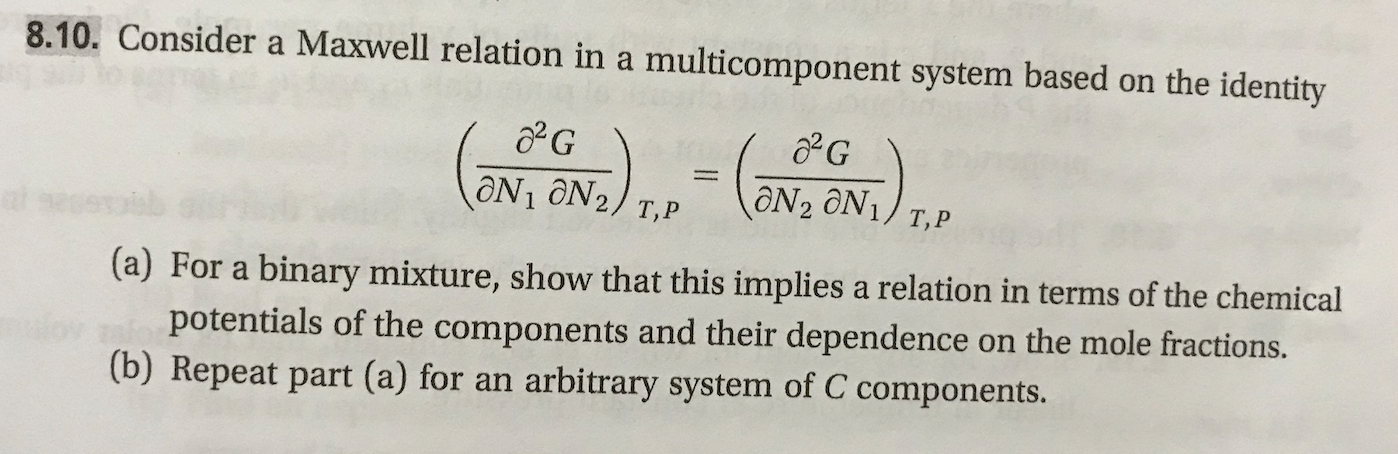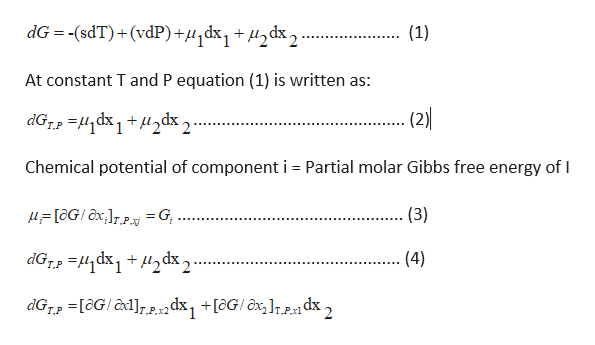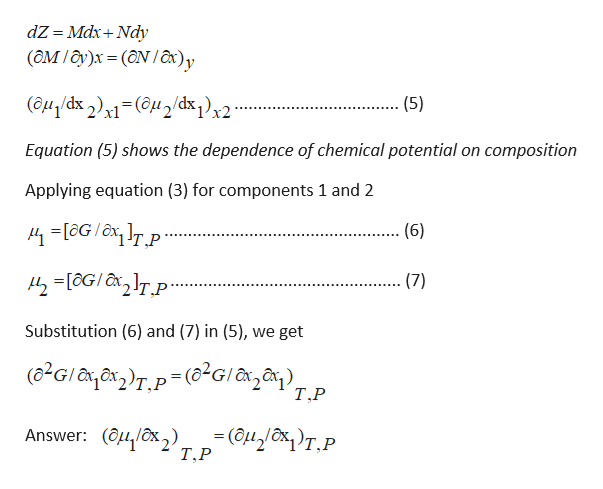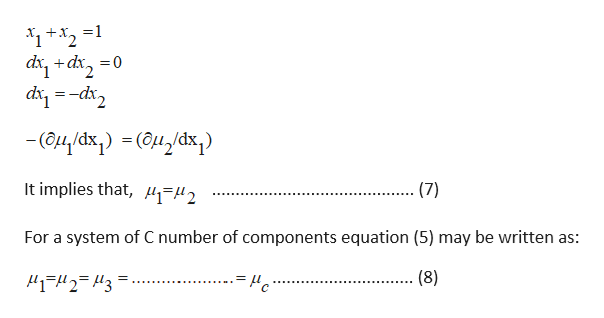# 8.10. Consider a Maxwell relation in amulticomponent system based on the identityaN2 ON1/ T,PON1 ON2/ T,Ps(a) For a binary mixture, show that this implies a relation in terms of the chemicalpotentials of the components and their dependence(b) Repeat part (a) for anon the mole fractions.arbitrary system of C components.

Question
74 views

This problem is (8.10) from a book  "Thermodynamics and Statistical Mechanics An Integrated Approach by M. Scott Shell"help_outlineImage Transcriptionclose8.10. Consider a Maxwell relation in a multicomponent system based on the identity aN2 ON1/ T,P ON1 ON2/ T,P s (a) For a binary mixture, show that this implies a relation in terms of the chemical potentials of the components and their dependence (b) Repeat part (a) for an on the mole fractions. arbitrary system of C components. fullscreen
check_circle

Step 1

For a system of variable composition (open system), the differential change in molar Gibbs free energy for 1 mol of a mixture/solution is written as:help_outlineImage TranscriptionclosedG (sdT)+(vdP)+u^dx1 + /t2dx2 (1) _ At constant T and P equation (1) is written as: (2) 7 хр^m+ 1 xp11- гоР Chemical potential of component i Partial molar Gibbs free energy of I aGIx = G (3) 7 хр^н + 1xp-1- гор dGr[aG/ x1]12 dx, +[aG/ x]r,,1 dx (4) 2 fullscreen
Step 2

Applying Euler reciprocity relation to equation (4), we get,help_outlineImage TranscriptionclosedZ = MdxNdy (ам /д)х %3D (ӘN /a)у (аn dx )- (@uzdx,)2 (5) Equation (5) shows the dependence of chemical potential on composition Applying equation (3) for components 1 and 2 -[oG/TP (6) 42oGITp. (7) Substitution (6) and (7) in (5), we get (82GITP(2GI, Т.Р Он бк), р (ануом)т.Р Answer: (4/x,) Т.Р fullscreen
Step 3

(b)  Equation (5) is modified and rearranged as follows:

...help_outlineImage Transcriptioncloseо-р+ р Typ-- Чp -(аи дӑх, ) - (өм дӑк) It implies that, 2 (7) For a system of C number of components equation (5) may be written as: (8) fullscreen

### Want to see the full answer?

See Solution

#### Want to see this answer and more?

Solutions are written by subject experts who are available 24/7. Questions are typically answered within 1 hour.*

See Solution
*Response times may vary by subject and question.
Tagged in

### Chemical Engineering